# Image Processing 101: Point Operations

This article is a continuation of our Image Processing 101 blog series:

Spatial domain image enhancement techniques can be divided into two broad categories:

• Point operations
• Neighborhood operations

This post discusses the point operations and the next post will be covering the neighborhood operations.

Point operations refer to running the same conversion operation for each pixel in a grayscale image. The transformation is based on the original pixel and is independent of its location or neighboring pixels.

Let “r” and “s” be the gray value at a point (x, y) of the input image f (x, y) and the output image g (x, y), then the point operation can be defined by the following formula.

s= T (r)

Where T is the point operator of a certain gray-level mapping relationship between the original image and the output image. Point operations are often used to change the grayscale range and distribution.

## Power-Law (Gamma) Transformation

The gamma transform, also known as exponential transformation or power transformation, is a commonly used grayscale non-linear transformation.

The mathematical expression of the gamma transformation is as follows: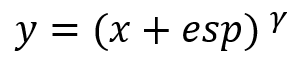Where x and y are the intensity level of a pixel in input and output, “esp” is the compensation coefficient, and γ is the gamma coefficient. When performing the conversion, it is a common practice to first convert the intensity level from the range of 0 to 255 to 0 to 1. Then perform the gamma conversion and at last restore to the original range.

When setting esp = 1, we can get a mapping form like the following, with the gray intensity of the input in the horizontal axis and the gray intensity of the output on the vertical axis.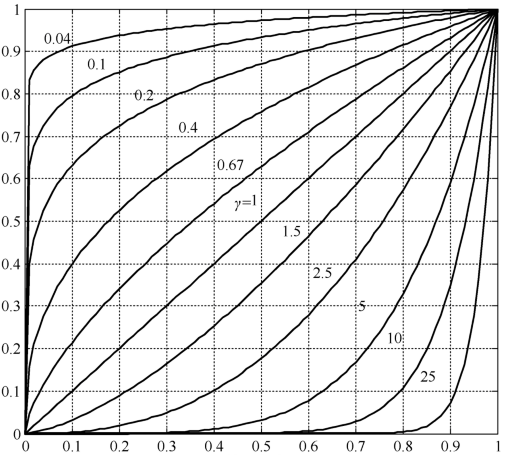Plots of the equation [formula] for various values of γ (c = 1 in all cases).

All curves were scaled to fit in the range shown. x-axis represents Input intensity level, r and y-axis represents the output Intensity Level S

The gamma transformation can selectively enhance the contrast of the dark region or the light region depending on the value of γ.

• When γ > 1, the contrast of the light gray area is enhanced. Take γ = 25 for example, the pixels with the range of 0.8-1 (at the scale of 256, it corresponds to 240-255) are mapped to the range of 0-1
• When γ < 1, the contrast of the dark gray area is enhanced
• When γ = 1, this transformation is linear, that is, the original image is not changed

## Grayscale Threshold Transform

Grayscale Threshold Transform converts a grayscale image into a black and white binary image. The user specifies a value that acts as a dividing line. If the gray value of a pixel is smaller than the dividing, the intensity of the pixel is set to 0, otherwise it’s set to 255. The value of the dividing line is called the threshold. The grayscale threshold transform is often referred to as thresholding, or binarization.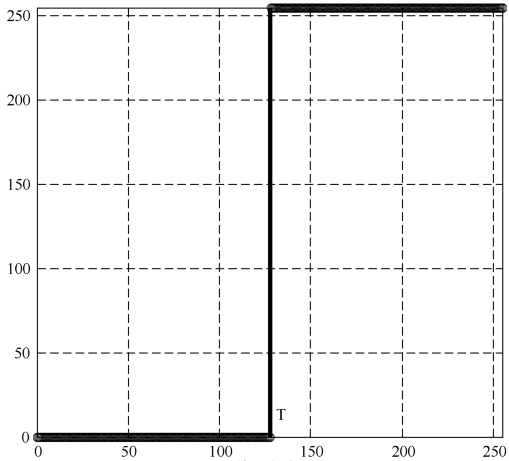## Contrast-Stretching Transformation

The goal of the contrast-stretching transformation is to enhance the contrast between different parts of an image, that is, enhances the gray contrast for areas of interest, and suppresses the gray contrast for areas that are not of interest.

Below are two possible functions: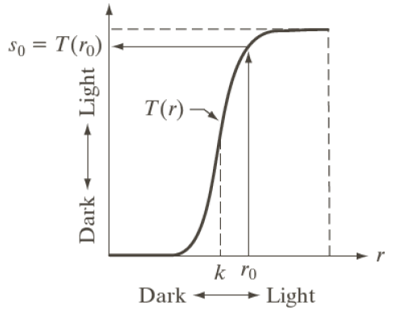If T(r) has the form as shown in the figure above, the effect of applying the transformation to every pixel to generate the corresponding pixels produce higher contrast than the original image, by:

• Darkening the levels below k in the original image
• Brightening the levels above k in the original image

Points (r1, s1) and (r2, s2) control the shape of the transformation. The selection of control points depends upon the types of image and varies from one image to another image. If r1 = s1 and r2 = s2 then the transformation is linear and this doesn’t affect the image. In other case we can calculate the intensity of output pixel, provided intensity of input pixel is x, as follows:

• For 0 <= x <= r1 output = s1 / r1 * x
• For r1 < x <= r2 output = ((s2 – s1)/(r2 – r1))*(x – r1) + s1
• For r2 < x <= L-1 output = ((L-1 – s2)/(L-1 – r2))*(x – r2) + s2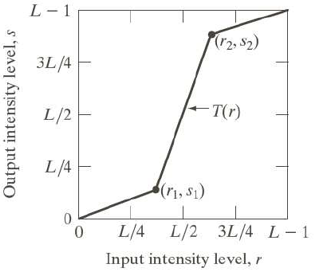## Histogram Equalization

Histogram equalization, also known as grayscale equalization, is a practical histogram correction technique.

It refers to the transformation where an output image has approximately the same number of pixels at each gray level, i.e., the histogram of the output is uniformly distributed. In the equalized image, the pixels will occupy as many gray levels as possible and be evenly distributed. Therefore, such image has a higher contrast ratio and a larger dynamic range.

Subscribe Newsletter

Subscribe to our mailing list to get the monthly update.

Subscribename@email.com